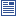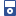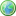Accessibility options:

# Logarithms resources

Show me all resources applicable to

### Community Project (1)Exponential and Logarithm for Economics and Business Studies (SOURCE)
Latex source, image files and metadata for the Fact & Formulae leaflet "Exponential and Logarithm for Economics and Business Studies" contributed to the mathcentre Community Project by Morgiane Richard (University of Aberdeen) and reviewed by Shazia Ahmed (University of Glasgow) and Anthony Cronin (University College Dublin).

### Facts & Formulae Leaflets (1)Exponential and Logarithm for Economics and Business Studies
Overview of the properties of the functions e and ln and their applications in Economics. This leaflet has been contributed to the mathcentre Community Project by Morgiane Richard (University of Aberdeen) and reviewed by Shazia Ahmed (University of Glasgow) and Anthony Cronin (University College Dublin).

### Guides (1)Just the Maths (A.J.Hobson)
"Just the Maths" authored by the late Tony Hobson, former Senior Lecturer in Mathematics of the School of Mathematical and Information Sciences at Coventry University, is a collection of separate mathematics units, in chronological topic-order, intended for foundation level and first year degree level in higher education where mathematics is a service discipline e.g. engineering.

### iPOD Video (10)Logarithms 1
Logarithms appear in all sorts of calculations in engineering and science, business and economics. Before the days of calculators they were used to assist in the process of multiplication by replacing the operation of multiplication by addition. Similarly, they enabled the operation of division to be replaced by subtraction. They remain important in other ways, one of which is that they provide the underlying theory of the logarithm function. This has applications in many fields, for example, the decibel scale in acoustics. This resource is released under a Creative Commons license Attribution-Non-Commercial-No Derivative Works and the copyright is held by Skillbank Solutions Ltd.Logarithms 10
Logarithms appear in all sorts of calculations in engineering and science, business and economics. Before the days of calculators they were used to assist in the process of multiplication by replacing the operation of multiplication by addition. Similarly, they enabled the operation of division to be replaced by subtraction. They remain important in other ways, one of which is that they provide the underlying theory of the logarithm function. This has applications in many fields, for example, the decibel scale in acoustics. This resource is released under a Creative Commons license Attribution-Non-Commercial-No Derivative Works and the copyright is held by Skillbank Solutions Ltd.Logarithms 2
Logarithms appear in all sorts of calculations in engineering and science, business and economics. Before the days of calculators they were used to assist in the process of multiplication by replacing the operation of multiplication by addition. Similarly, they enabled the operation of division to be replaced by subtraction. They remain important in other ways, one of which is that they provide the underlying theory of the logarithm function. This has applications in many fields, for example, the decibel scale in acoustics. This resource is released under a Creative Commons license Attribution-Non-Commercial-No Derivative Works and the copyright is held by Skillbank Solutions Ltd.Logarithms 3
Logarithms appear in all sorts of calculations in engineering and science, business and economics. Before the days of calculators they were used to assist in the process of multiplication by replacing the operation of multiplication by addition. Similarly, they enabled the operation of division to be replaced by subtraction. They remain important in other ways, one of which is that they provide the underlying theory of the logarithm function. This has applications in many fields, for example, the decibel scale in acoustics. This resource is released under a Creative Commons license Attribution-Non-Commercial-No Derivative Works and the copyright is held by Skillbank Solutions Ltd.Logarithms 4
Logarithms appear in all sorts of calculations in engineering and science, business and economics. Before the days of calculators they were used to assist in the process of multiplication by replacing the operation of multiplication by addition. Similarly, they enabled the operation of division to be replaced by subtraction. They remain important in other ways, one of which is that they provide the underlying theory of the logarithm function. This has applications in many fields, for example, the decibel scale in acoustics. This resource is released under a Creative Commons license Attribution-Non-Commercial-No Derivative Works and the copyright is held by Skillbank Solutions Ltd.Logarithms 5
Logarithms appear in all sorts of calculations in engineering and science, business and economics. Before the days of calculators they were used to assist in the process of multiplication by replacing the operation of multiplication by addition. Similarly, they enabled the operation of division to be replaced by subtraction. They remain important in other ways, one of which is that they provide the underlying theory of the logarithm function. This has applications in many fields, for example, the decibel scale in acoustics. This resource is released under a Creative Commons license Attribution-Non-Commercial-No Derivative Works and the copyright is held by Skillbank Solutions Ltd.Logarithms 6
Logarithms appear in all sorts of calculations in engineering and science, business and economics. Before the days of calculators they were used to assist in the process of multiplication by replacing the operation of multiplication by addition. Similarly, they enabled the operation of division to be replaced by subtraction. They remain important in other ways, one of which is that they provide the underlying theory of the logarithm function. This has applications in many fields, for example, the decibel scale in acoustics. This resource is released under a Creative Commons license Attribution-Non-Commercial-No Derivative Works and the copyright is held by Skillbank Solutions Ltd.Logarithms 7
Logarithms appear in all sorts of calculations in engineering and science, business and economics. Before the days of calculators they were used to assist in the process of multiplication by replacing the operation of multiplication by addition. Similarly, they enabled the operation of division to be replaced by subtraction. They remain important in other ways, one of which is that they provide the underlying theory of the logarithm function. This has applications in many fields, for example, the decibel scale in acoustics. This resource is released under a Creative Commons license Attribution-Non-Commercial-No Derivative Works and the copyright is held by Skillbank Solutions Ltd.Logarithms 8
Logarithms appear in all sorts of calculations in engineering and science, business and economics. Before the days of calculators they were used to assist in the process of multiplication by replacing the operation of multiplication by addition. Similarly, they enabled the operation of division to be replaced by subtraction. They remain important in other ways, one of which is that they provide the underlying theory of the logarithm function. This has applications in many fields, for example, the decibel scale in acoustics. This resource is released under a Creative Commons license Attribution-Non-Commercial-No Derivative Works and the copyright is held by Skillbank Solutions Ltd.Logarithms 9
Logarithms appear in all sorts of calculations in engineering and science, business and economics. Before the days of calculators they were used to assist in the process of multiplication by replacing the operation of multiplication by addition. Similarly, they enabled the operation of division to be replaced by subtraction. They remain important in other ways, one of which is that they provide the underlying theory of the logarithm function. This has applications in many fields, for example, the decibel scale in acoustics. This resource is released under a Creative Commons license Attribution-Non-Commercial-No Derivative Works and the copyright is held by Skillbank Solutions Ltd.

### Quick Reference (12)Indicial equations
An indicial equation is one in which the power is unknown. Such equations often occur in the calculation of compound interest.Logarithms
We use logarithms to write expressions involving powers in a different form. If you can work confidently with powers, you should have no problems handling logarithmsLogarithms - changing the base
Sometimes it is necessary to find logs to bases other then 10 and e. There is a formula which enables us to do this. This leaflet states and illustrates the use of this formula.Solving equations involving logarithms and exponentials
This leaflet shows how simple equations involving logarithms or exponentials can be solved. (Engineering Maths First Aid Kit 3.8)Solving equations using logs
Logs can be used to solve equations when the unknown occurs as a power. This leaflet explains how.The exponential constant e
The letter e is used in many mathematical calculations to stand for a particular number known as the exponential constant. This leaflet provides information about this important constant, and the related exponential function.The laws of logarithms
This leaflet explains and illustrates the laws governing the manipulation of logarithms. (Engineering Maths First Aid Kit 2.20)The laws of logarithms
There are rules, or laws, which are used to rewrite expressions involving logs in different forms. This leaflet states and illustrates these rules.The laws of logarithms
There are a number of rules known as the laws of logarithms. These allow expressions involving logarithms to be rewritten in a variety of different ways. The laws apply to logarithms of any base, but the same base must be used throughout a calculation.The logarithm function
This leaflet provides a table of values and a graph of the logarithm function. (Engineering Maths First Aid Kit 3.7)What is a logarithm ?
This leaflet explains logarithms. (Engineering Maths First Aid Kit 2.19)What is a logarithm ?
Logarithms can be used to write expressions involving powers in alternative forms. This leaflet explains how.

### Teach Yourself (1)Logarithms
This booklet explains what is meant by a logarithm. It states and illustrates the laws of llogarithms. It explains the standard bases 10 and e. Finally it shows how logarithms can be used to solve certain types of equations.

### Test Yourself (3)Logarithms and solving equations - Numbas
8 questions using logarithms. 7 questions use logarithms to solve equations. Numbas resources have been made available under a Creative Commons licence by the School of Mathematics & Statistics at Newcastle University.Logarithms and solving equations - Numbas
8 questions using logarithms. Of these 7 questions use logarithms to solve equations.Maths EG
Computer-aided assessment of maths, stats and numeracy from GCSE to undergraduate level 2. These resources have been made available under a Creative Common licence by Martin Greenhow and Abdulrahman Kamavi, Brunel University.

### Third Party Resources (2)Mathematics Support Materials from the University of Plymouth
Support material from the University of Plymouth:
The output from this project is a library of portable, interactive, web based support packages to help students learn various mathematical ideas and techniques and to support classroom teaching.
There are support materials on ALGEBRA, GRAPHS, CALCULUS, and much more.
This material is offered through the mathcentre site courtesy of Dr Martin Lavelle and Dr Robin Horan from the University of Plymouth.University of East Anglia (UEA) Interactive Mathematics and Statistics Resources
The Learning Enhancement Team at the University of East Anglia (UEA) has developed la series of interactive resources accessible via Prezi mind maps : Steps into Numeracy, Steps into Algebra, Steps into Trigonometry, Bridging between Algebra and Calculus, Steps into Calculus, Steps into Differential Equations, Steps into Statistics and Other Essential Skills.

### Video (1)Logarithms
Logarithms appear in all sorts of calculations in engineering and science, business and economics. Before the days of calculators they were used to assist in the process of multiplication by replacing the operation of multiplication by addition. Similarly, they enabled the operation of division to be replaced by subtraction. They remain important in other ways, one of which is that they provide the underlying theory of the logarithm function. This has applications in many fields, for example, the decibel scale in acoustics. (Mathtutor Video Tutorial) This resource is released under a Creative Commons license Attribution-Non-Commercial-No Derivative Works and the copyright is held by Skillbank Solutions Ltd.

### Video with captions which require edits (1)Logarithms
Logarithms appear in all sorts of calculations in engineering and science, business and economics. Before the days of calculators they were used to assist in the process of multiplication by replacing the operation of multiplication by addition. Similarly, they enabled the operation of division to be replaced by subtraction. They remain important in other ways, one of which is that they provide the underlying theory of the logarithm function. This has applications in many fields, for example, the decibel scale in acoustics. (Mathtutor Video Tutorial) The video is released under a Creative Commons license Attribution-Non-Commercial-No Derivative Works and the copyright is held by Skillbank Solutions Ltd.